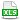HomeTechniques and Tips@RISK Simulation: Numerical ResultsMode of Continuous Data

# 6.9. Mode of Continuous Data

Applies to: @RISK, all versions

I'm displaying the mode of my data, and it seems to be very far from the tallest bar in the histogram. What is wrong? How does @RISK compute the mode of a continuous distribution?

The traditional definition of the mode of discrete data is the most frequently occurring value of the variable. An analogous definition works well for most theoretical continuous distributions: you have a smooth probability density curve pdf(x), and the mode is simply the value of x where the pdf(x) is highest. But for continuous data in simulation results, it's unusual to have identical data points, and therefore a new definition is needed.

Different authorities use different definitions and therefore find different modes; the way you bin the data can also change which value you call the mode. One way to come up with a mode is to divide the n simulated data points into k bins, each with n/k consecutive data points, and then look at the widths of the bins. The narrowest bin is the one where the points are clustered closest together, which means that the probability density is greatest in that bin, so the mode must be there.

@RISK uses that method. It divides the simulated data into k = 100 bins unless there are fewer than 300 data points in the simulation; in that case @RISK uses k = n/3 bins, so that a bin never has fewer than three points. @RISK then finds the narrowest bin, where the points are most closely clustered together.  Finally, it computes the mean value of the n/k points in that bin, and reports that value as the mode. (This information is current as of 2015-05-01, but may change in a future release of @RISK.)

The binning for purposes of finding the mode is almost always different from the binning for a histogram of the data in Browse Results or other graphs. Even if you specify a histogram of 100 bins, they will still be different from the histogram bins. When @RISK is finding a mode, the bins all contain the same number of points and have different widths. On the histogram, the bins (bars) all have the same width and contain different numbers of points. This is how the mode can be far from the tallest bar in the histogram.

A simple example is attached. That example does show how changing the graph settings can reveal the mode, but that technique won't work on every distribution.

By the way, when you do distribution fitting, @RISK uses this same computation. That's how it gets the approximate mode of your sample data that it shows in the fit results window, for comparison with the fitted distribution. This computation is not used in any way in the process of fitting distributions to your data; it's purely for display of the comparison.

Last edited: 2015-05-01

•ModeOfContinuous.xls